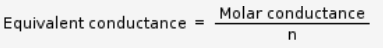# What is the formula for Equivalent conductivity and how is it different from molar conductivity formula?

What is the formula for Equivalent conductivity and how is it different from molar conductivity formula??

Equivalent conductance is defined as the conductance of all the ions produced by one gram equivalent of an electrolyte in a given solution. Equivalent conductance λ = k × V Where V is the volume in mL containing 1 g equivalent of the electrolyte. Molar conductance is defined as the conductance of all the ions produced by ionization of 1 g mole of an electrolyte when present in V mL of solution. It is denoted by . Molar conductance μ = k ×V Where V is the volume in mL containing 1 g mole of the electrolyte. If c is the concentration of the solution in g mole per litre, thenWhereEquivalent conductance is defined as the conductance of all the ions produced by one gram equivalent of an electrolyte in a given solution.

Equivalent conductance λ = k × V

Where V is the volume in mL containing 1 g equivalent of the electrolyte.

Molar conductance is defined as the conductance of all the ions produced by ionization of 1 g mole of an electrolyte when present in V mL of solution. It is denoted by .

Molar conductance μ = k ×V

Where V is the volume in mL containing 1 g mole of the electrolyte. If c is the concentration of the solution in g mole per litre, then Where